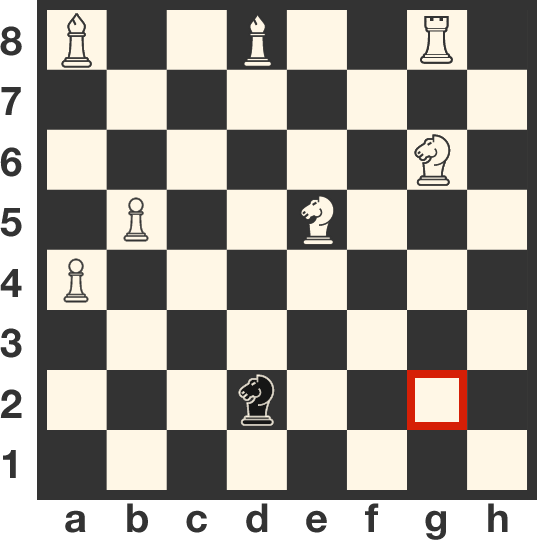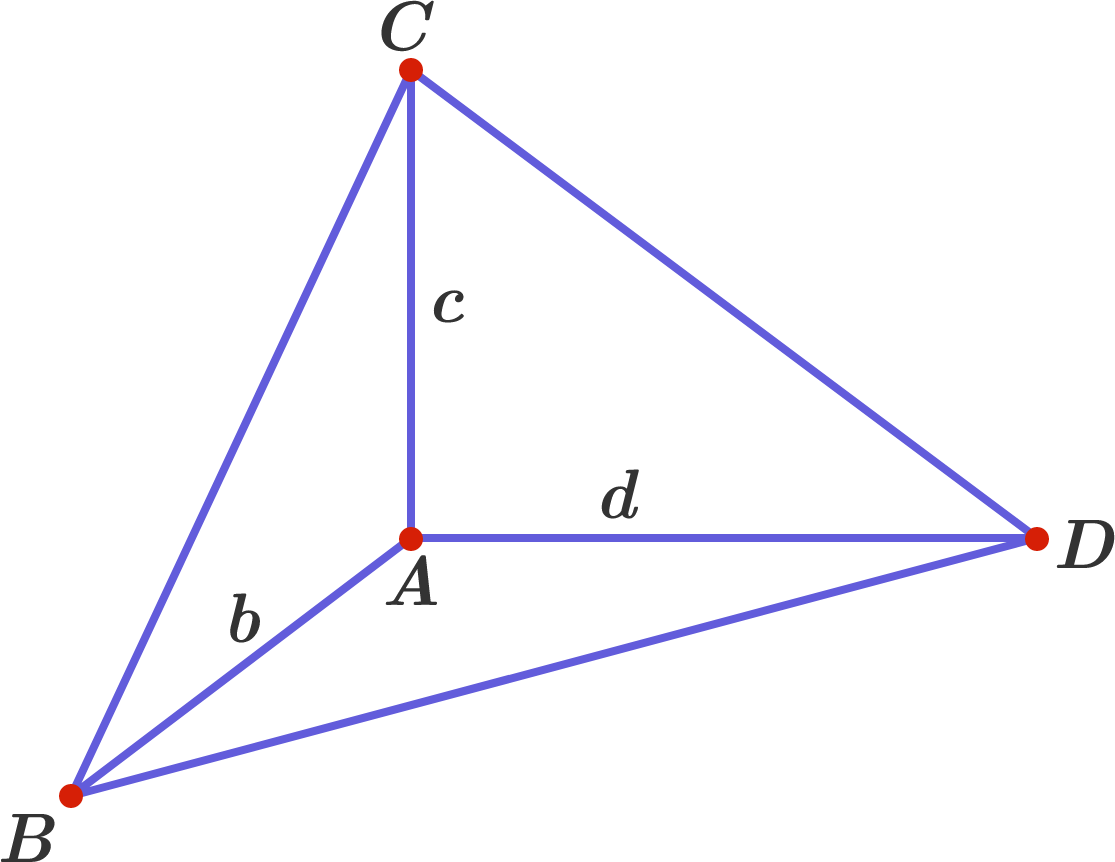# Problems of the Week

Contribute a problem

$a,b,$ and $c$ are real numbers such that $\frac{1}{a}+\frac{1}{b}+\frac{1}{c}=\frac{1}{a+b+c}.$ Does the following equation hold for all odd integers $n?$ $\frac{1}{a^n}+\frac{1}{b^n}+\frac{1}{c^n}=\frac{1}{a^n+b^n+c^n}$

The black knight on d2 has to reach g2 safely – so that no white pieces can capture it along the way or when it reaches its destination. What is the minimum number of moves required for the black knight to accomplish this?Details and Assumptions:

• White will only move a piece to capture the black knight. White will skip its turn otherwise.
• The black knight can capture white pieces.

Consider a circle with a starting circumference of $C_0 = 100$ units. Suppose you start on a point on that circle and proceed to walk along it until you take your first step on or past your starting position. Given the rules below:

• each step you take is worth 1 unit;
• for each step you take, the circumference of the circle is scaled by the formula $C_s = C_0\times (s+1)$, where $s$ is the number of steps made (so after the first step the circumference is now 200, after the second step the circumference is 300, etc.),

which of the following gives the best (i.e. most accurate) approximation to the number of steps needed to complete the task?


Note: If you think that the task will never be completed, then answer $\infty$. Also, the $\left \lfloor x \right \rfloor$ is the usual floor function.

The length $(L)$ and width $(W)$ of a rectangle are random variables, independently and uniformly distributed on the interval $[9,11],$ and the area $(A)$ of the rectangle is also a random variable: $L,W \sim U(9,11);\ \ \ \ A = LW.$ It is not difficult to see that the expected value of the area is $E[A] = 100.$

Determine the median of the area.

$ABCD$ is a tetrahedron where all three face angles at vertex $A$ are right angles and the side lengths $AB = b, AC = c, AD = d$ are all distinct, positive integers.

What is the smallest possible integer value for the area of $\triangle BCD?$×